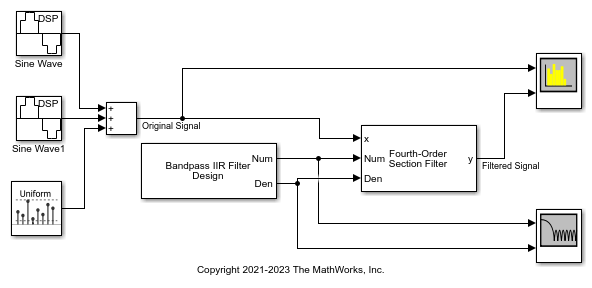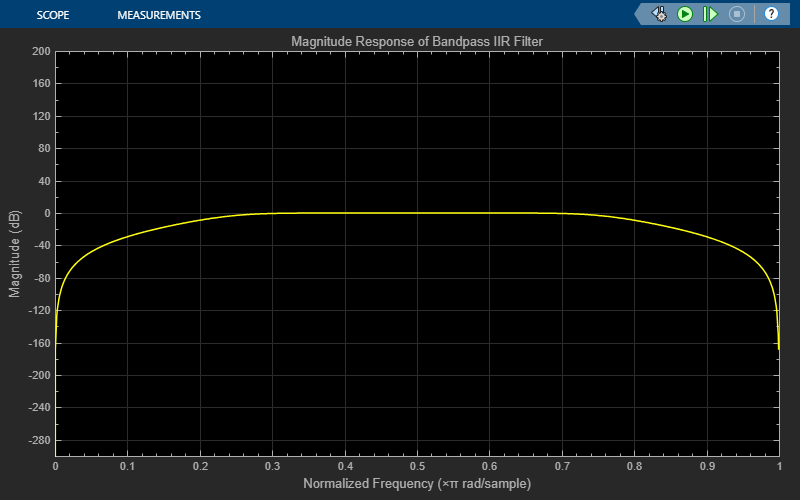# Filter Noisy Signal Using Fourth-Order Section (FOS) Filter in Simulink

Filter a noisy sinusoidal signal using the Fourth-Order Section Filter block. Visualize the original signal and the filtered signal using a Spectrum Analyzer.

Open and run the model.

The input signal is a sum of two sine waves with the frequencies of 100 Hz and 350 Hz. The sample rate is 1000 Hz and the number of samples in each frame is 1024. Add zero-mean white Gaussian noise with a variance of 1e-4 to the sum of the sine waves.

The numerator and denominator coefficients of the fourth-order section filter are obtained using the `designParamEq` function from the Audio Toolbox™.

The following code is used in the model to generate the coefficients.

```N = [2,4]; gain = [5,10]; centerFreq = [0.025,0.75]; bandwidth = [0.025,0.35]; mode = "fos"; [num,den] = designParamEQ(N,gain,centerFreq,bandwidth,mode,Orientation="row"); ```

Filter the noisy sinusoidal signal using the Fourth-Order Section Filter filter block. Visualize the original sinusoidal signal and the filtered signal using the Spectrum Analyzer.